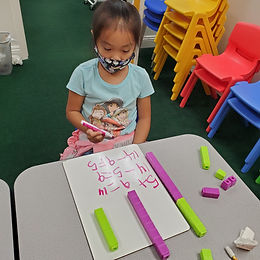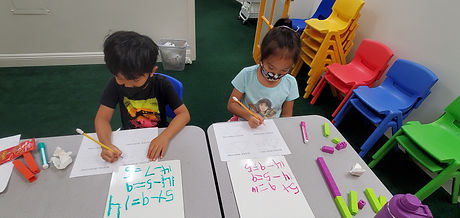## Ms. Raejean

### Target 1​

###### Lesson Type:

Continuation

Number Operation

:

Number Meaning

Match number and quantity for numbers zero to 100.

###### 1:

Develop strategies of grouping quantities to count larger quantities faster (i.e. base-ten blocks).

2nd

###### Vocabulary:

Quick Images, Grouping, Pattern

Activities:

Students continued to work on seeing patterns and grouping items to count them faster. Quick images were shown, and students verbalized how they counted the number of dots. Sometimes each dot was counted, and other times, groups were recognized. Ex. 4 and 5 dots make 9 altogether. Using dice to show the image that was seen helped to identify the grouping and total amount of dots. Ex. 3 & 6 dots make 9 altogther.### Home Exploration

###### Guiding Questions:## Absent Students:

### Target 2

:

###### 1:

Identify the Commutative Property of Addition.

###### 2:

Understand that changing the order of addends, does not change the sum.

2nd

###### Vocabulary:

Activities:

The term "commutative property" was introduced. We reviewed how changing the order of the addends doesn't change the sum (answer). Students matched the equations to show the commutative property. Ex. 3 + 8 = 8 + 3. We also reviewed how starting with the bigger number and adding on the smaller number made it easier to add. Ex. start with 8 & add 3 more, so 8...9, 10, 11. Students solved the equations that started with the bigger number, which allowed them to practice "adding on."### Home Exploration

###### Guiding Questions:### Target 3

:

###### 1:

Understand the inverse relationship between addition and subtraction.

2nd

###### Vocabulary:

Inverse Operations, Fact Family

Activities:

The term "Inverse Operations" was introduced. Students used the same numbers in the equation, but in a different order, and subtracted from one another. Using the inverse operation helps students double check their work. Students used unifix cubes to represent the 2 addends and the sum. Ex. 5 green + 9 purple = 14 altogether. Then the numbers were swapped. 14 altogether - 9 purple = 5 green. OR 14 altogether - 5 green = 9 purple. We used a "Fact Family" triangle to show the relationship between the numbers. 14 goes at the top, and 5 & 9 go on the bottom. This helped students visualize that 5 + 9 = 14 and 9 + 5 = 14; while 14 - 9 = 5 and 14 - 5 = 9. We will continue to explore the inverse relationship between addition and subtraction.### Home Exploration